## NAME

v.buffer - Creates a buffer around vector features of given type.

## KEYWORDS

vector, buffer, area, circle, geometry, line, grow, shrink

## SYNOPSIS

v.buffer
v.buffer --help
v.buffer [-sct] input=name [layer=string] [cats=range] [where=sql_query] [type=string[,string,...]] output=name [distance=float] [minordistance=float] [angle=float] [column=name] [scale=float] [tolerance=float] [--overwrite] [--help] [--verbose] [--quiet] [--ui]

### Flags:

-s
Make outside corners straight
-c
Do not make caps at the ends of polylines
-t
Transfer categories and attributes
--overwrite
Allow output files to overwrite existing files
--help
Print usage summary
--verbose
Verbose module output
--quiet
Quiet module output
--ui
Force launching GUI dialog

### Parameters:

input=name [required]
Name of input vector map
Or data source for direct OGR access
layer=string
Layer number or name ('-1' for all layers)
A single vector map can be connected to multiple database tables. This number determines which table to use. When used with direct OGR access this is the layer name.
Default: -1
cats=range
Category values
Example: 1,3,7-9,13
where=sql_query
WHERE conditions of SQL statement without 'where' keyword
Example: income < 1000 and population >= 10000
type=string[,string,...]
Input feature type
Options: point, line, boundary, centroid, area
Default: point,line,area
output=name [required]
Name for output vector map
distance=float
Buffer distance along major axis in map units
minordistance=float
Buffer distance along minor axis in map units
angle=float
Angle of major axis in degrees
Default: 0
column=name
Name of column to use for buffer distances
scale=float
Scaling factor for attribute column values
Default: 1.0
tolerance=float
Maximum distance between theoretical arc and polygon segments as multiple of buffer (default 0.01)

## DESCRIPTION

v.buffer creates a buffer around features of given type, which have a category in the given layer. The tolerance controls the number of vector segments being generated (the smaller the value, the more vector segments are generated).

## NOTES

Internal buffers for areas can be generated with negative distance values ("inward buffer" or "negative buffer" or "shrinking").

v.buffer fusions the geometries of buffers by default. Categories and attribute table will not be transferred (this would not make sense as one buffer geometry can be the result of many different input geometries). To transfer the categories and attributes the user can set the t flag. This will result in buffers being cut up where buffers of individual input geometries overlap. Each part that is the result of overlapping buffers of multiple geometries will have multiple categories corresponding to those geometries, and multiple buffer areas can have the same category. The buffer for the input feature with category X can thus be retrieved by selecting all buffer areas with category X (see example below).

Buffers for lines and areas are generated using the algorithms from the GEOS library.

For advanced users: built-in buffer algorithm no longer desired, we use GEOS: If GRASS is not compiled with GEOS support or environmental variable GRASS_VECTOR_BUFFER is defined, then GRASS generates buffers using built-in buffering algorithm (which is still buggy for some input data).

The options minordistance, angle, tolerance are kept for backward compatibility and have no effect with GEOS buffering.

### Corner settings

The following vector line related corners (also called "cap") exist:
• no cap: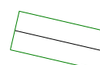• rounded cap: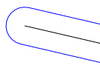• square cap: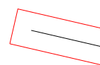By default v.buffer creates rounded buffers (blue color on figure below):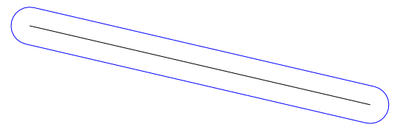Straight corners with caps are created by -s flag (red color on the figure below), while -c flag doesn't make caps at the ends of polylines (green color on the figure below):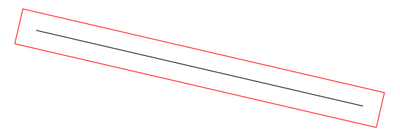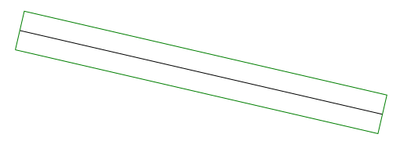Using -s with a point vector map as input data, square buffers are created instead of round buffers.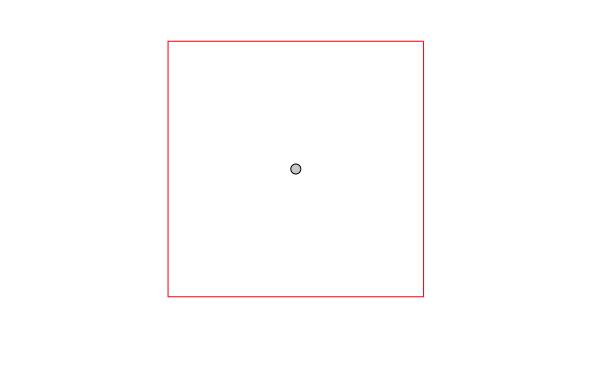## EXAMPLES

All examples are based on the North Carolina sample dataset.

### Buffer around input lines

```v.buffer input=roadsmajor output=roadsmajor_buffer type=line distance=100
```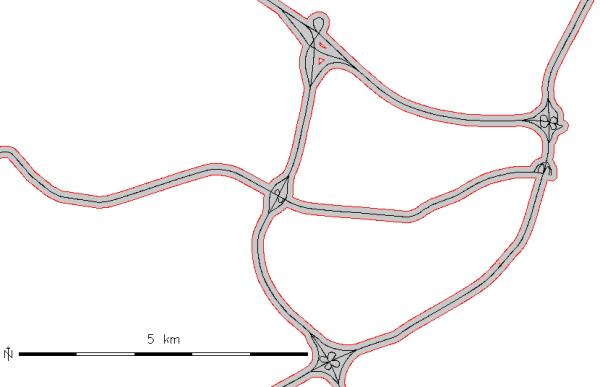Buffer of 100m along the "roadsmajor" lines (map subset, original center line shown in black)

### Circles around input points

```v.buffer input=hospitals output=hospitals_circled type=point distance=2000
```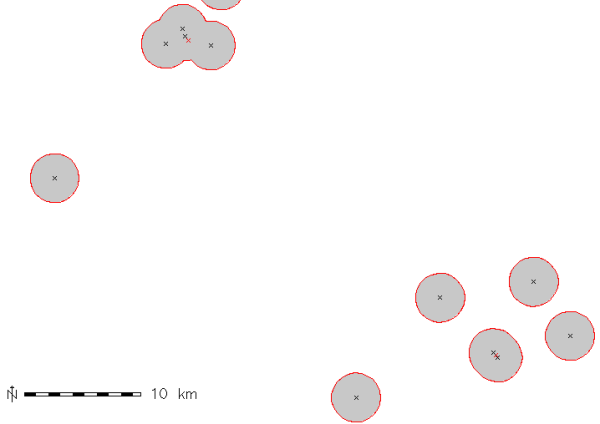Buffer of 2000m around the "hospitals" points (map subset, original points shown in black, new area centroids in red)

### Circles around input points with attribute transfer

```v.buffer input=hospitals output=hospitals_circled type=point distance=1000 -t

# display buffer around hospital with category 36,
# this buffer is composed of several areas:
d.vect map=hospitals_circled type=area layer=1 cats=36
# extract this buffer, dissolving boundaries
v.extract in=hospitals_circled output=hospital_36_circled layer=1 cats=36 -d
```

### Buffer around input areas

```v.buffer input=lakes output=lakes_buffer type=area distance=100
```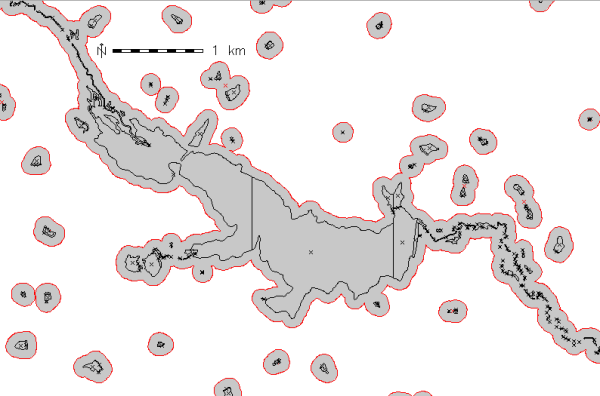Buffer of 100m around the "lakes" polygons (map subset, original areas shown in black)

### Buffer inside input areas

In this example, an internal buffer ("inward buffer" or "negative buffer") is generated using a negative distance value:
```v.buffer input=lakes output=lakes_buffer type=area distance=-50
```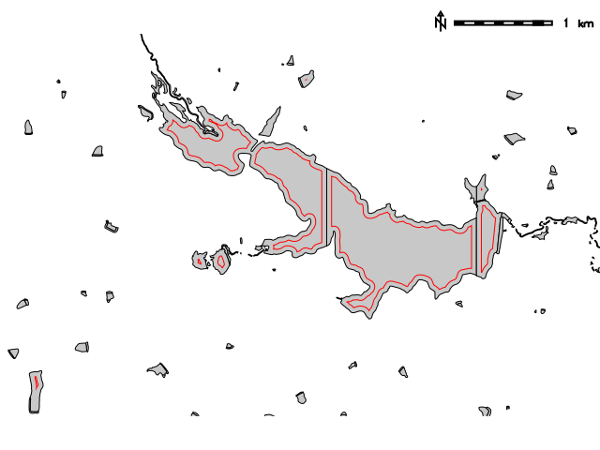Internal buffer of 50m inside the "lakes" polygons (map subset, original areas shown in black)
Not all features are buffered, only the polygons that allow creation inside a topological cleaned polygon.

## REFERENCES

r.buffer, v.parallel, v.extract, v.type, v.patch, v.db.connect